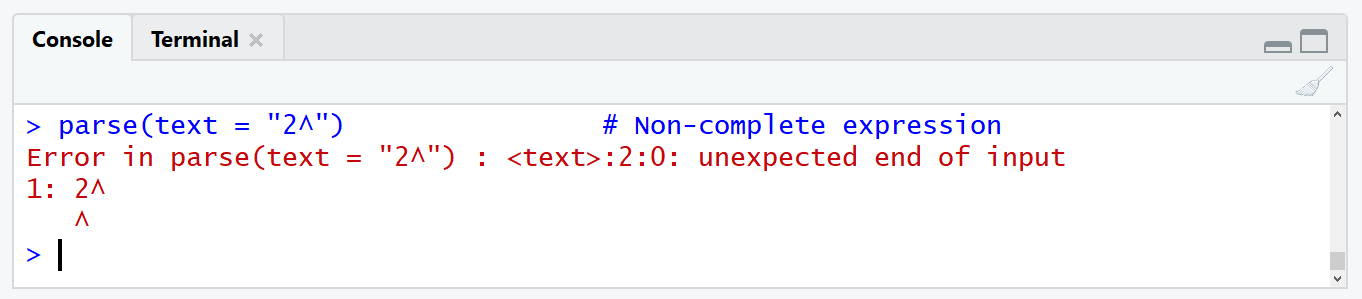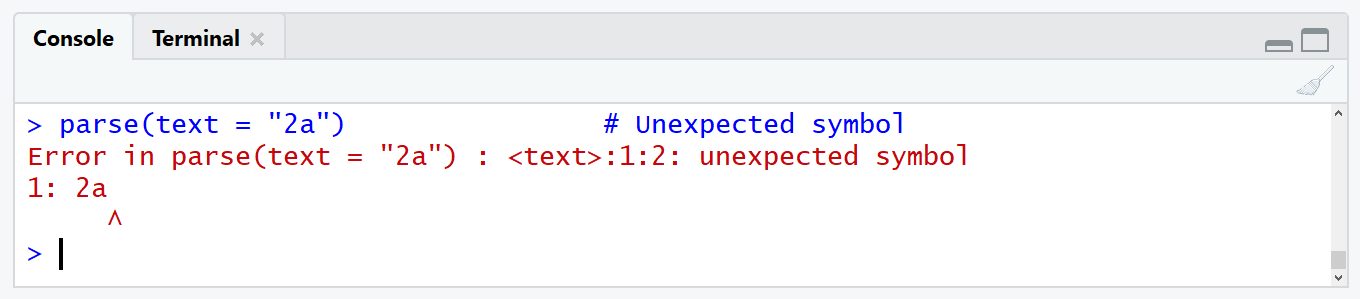# parse, deparse & expression Functions in R | Handle Character String & Expression

In this tutorial I will show you how to create expressions, how to convert characters to expressions, and how to convert expressions to characters.

This tutorial is based on the expression, parse, and deparse R programming functions. So let’s first have a look at the basic R syntax and the definitions of the functions:

Basic R Syntax:

```expression(character)
parse(text = character)
deparse(expression)```

Definition:

The expression function creates an R object of the expression class.

The parse function converts an R object of the character class to an R object of the expression class.

The deparse function converts an R object of the expression class to an R object of the character class.

In the following article, I will show you five examples for expression, parse, and deparse in R. Let’s dive right in!

## Example 1: Create R Expression (expression Function)

In the first example, I’ll show you how to create an R expression with the expression function:

```x1 <- expression(2^2)             # Create expression in R
x1                                # Print output to RStudio console
# expression(2^2)```

With the previous R code, we have created the expression 2^2 and have stored this expression in the data object x1. We can double check the class of this data object with the class function:

```class(x1)                         # Check class of example data
# "expression"```

Looks good! We could now evaluate this expression with the eval function:

```eval(x1)                          # Use eval function to evaluate expression
# 4```

2^2 = 4… Correct!

However, the eval function is not the scope of this tutorial. If you want to learn more about eval in R, check out this tutorial.

## Example 2: Convert Character String to R Expression (parse Function)

It happens quite often that we have an expression, which is stored as character class in R. If we want to evaluate such an expression, we need to convert our data object from character class to expression class.

I’ll show an example. Let’s first create an exemplifying data object:

```x2 <- "2^2"                       # Create character string
x2                                # Print output to RStudio console
# "2^2"```

As you can see based on the class function, our example data has the class character:

```class(x2)                         # Check class of example data
# "character"```

If we want to convert this character string to an expression, we can use the parse function:

```x2 <- parse(text = x2)            # Convert character to expression
x2                                # Print output to RStudio console
# expression(2^2)```

Let’s check the class of our data again:

```class(x2)                         # Check class of example data
# "expression"```

The data object x2 is an expression now and can be evaluated with the eval function (as shown in Example 1).

## Example 3: Convert Expression String to Character (deparse Function)

We can also do the conversion of Example 2 in the opposite way (i.e. converting from expression to character class). For this example, I’m going to use the data object x2, that we have converted to an expression in Example 2.

With the deparse function, we can convert this R expression back to the character class and store it in the new data object x3:

```x3 <- deparse(x2)                 # Convert expression to character
x3                                # Print output to RStudio console
# "structure(expression(2^2), srcfile = <environment>, wholeSrcref = structure(c(1L, " "0L, 2L, 0L, 0L, 0L, 1L, 2L), srcfile = <environment>, class = \"srcref\"))"```

Let’s check the result with the class function:

```class(x3)                         # Check class of example data
# "character"```

x3 is a character string – looks good.

Note that this character string is not the same as our original input object x2 (as created in Example 2), since it contains additional information on the expression. For instance, this can be useful to create informative labels for data sets and plots.

## Typical Parsing Errors & How to Avoid Them

If the expression, parse, and deparse functions are not applied correctly, they might lead to errors. In the following two examples, I’m going to show you two typical errors that occur after applying the parse function.

### R parse Function Error: Unexpected End of Input

A typical error of the parse function is the following:

`parse(text = "2^")                # Non-complete expression`Figure 1: Parsing Error in R: Unexpected End of Input.

This error usually occurs when the expression does not end with a number or a letter. In this specific case our fake expression ends with a minus sign (i.e. -) and therefore the parse function returns an error to the RStudio console.

### R parse Function Error: Unexpected Symbol

Another typical error of the parse function is the following:

`parse(text = "2a")                # Unexpected symbol`Figure 2: Parsing Error in R: Unexpected Symbol.

This error occurs when several symbols are connected in an invalid way. In this specific case we tried to convert 2a to an expression without specifying if we want to do with these two values. Do we want to multiply, do we want to subtract…? We have to replace 2a with something like 2*a or 2-a in order to make the parse function work.

## Video Examples: Regular Expressions in R

In this tutorial I explained a lot about expressions in R. If you want to learn more about expressions in general, I can recommend the following video of Roger Peng’s YouTube channel. In the video, he explains how to use regular expressions in R.

Enjoy the video and let me know in the comments, in case you have any questions on the handling of character strings and expressions in R.

Please accept YouTube cookies to play this video. By accepting you will be accessing content from YouTube, a service provided by an external third party.If you accept this notice, your choice will be saved and the page will refresh.

Subscribe to the Statistics Globe Newsletter

•I.M.
May 8, 2022 11:37 am

Thank you! The mentioning of the `eval()` function was exactly what I was looking for!

•May 9, 2022 6:14 am